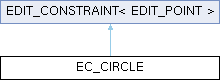KiCad PCB EDA Suite
EC_CIRCLE Class Reference

EDIT_CONSTRAINT that imposes a constraint that a point has to lie on a circle. More...

`#include <edit_constraints.h>`

Inheritance diagram for EC_CIRCLE:## Public Member Functions

EC_CIRCLE (EDIT_POINT &aConstrained, const EDIT_POINT &aCenter, const EDIT_POINT &aEnd)
Correct coordinates of the constrained edit handle. More...

virtual void Apply (EDIT_POINT &aHandle) override
Correct coordinates of the constrained edit handle. More...

void Apply ()
Correct coordinates of the constrained edit handle. More...

## Protected Attributes

EDIT_POINTm_constrained
Point that is constrained by rules implemented by Apply(). More...

## Private Attributes

const EDIT_POINTm_center
< Point that imposes the constraint (center of the circle). More...

const EDIT_POINTm_end

## Detailed Description

EDIT_CONSTRAINT that imposes a constraint that a point has to lie on a circle.

Definition at line 169 of file edit_constraints.h.

## ◆ EC_CIRCLE()

 EC_CIRCLE::EC_CIRCLE ( EDIT_POINT & aConstrained, const EDIT_POINT & aCenter, const EDIT_POINT & aEnd )
inline

Correct coordinates of the constrained edit handle.

Parameters
 aConstrained is the point that is put under constrain. aCenter is the point that is the center of the circle. aEnd is the point that decides on the radius of the circle.

Definition at line 177 of file edit_constraints.h.

## ◆ Apply() [1/2]

 void EDIT_CONSTRAINT< EDIT_POINT >::Apply ( )
inlineinherited

Correct coordinates of the constrained edit handle.

Definition at line 71 of file edit_constraints.h.

72  {
74  }
void Apply()
Correct coordinates of the constrained edit handle.
EDIT_POINT & m_constrained
Point that is constrained by rules implemented by Apply().

## ◆ Apply() [2/2]

 void EC_CIRCLE::Apply ( EDIT_POINT & aHandle )
overridevirtual

Correct coordinates of the constrained edit handle.

Implements EDIT_CONSTRAINT< EDIT_POINT >.

Definition at line 80 of file edit_constraints.cpp.

81 {
82  VECTOR2I centerToEnd = m_end.GetPosition() - m_center.GetPosition();
83  VECTOR2I centerToPoint = aHandle.GetPosition() - m_center.GetPosition();
84
86  double angle = centerToPoint.Angle();
87
88  VECTOR2I newLine( radius, 0 );
89  newLine = newLine.Rotate( angle );
90
91  aHandle.SetPosition( m_center.GetPosition() + newLine );
92 }
const EDIT_POINT & m_center
< Point that imposes the constraint (center of the circle).
virtual VECTOR2I GetPosition() const
Return coordinates of an EDIT_POINT.
Definition: edit_points.h:70
const EDIT_POINT & m_end
double Angle() const
Compute the angle of the vector.
Definition: vector2d.h:307
static DIRECTION_45::AngleType angle(const VECTOR2I &a, const VECTOR2I &b)
T EuclideanNorm() const
Compute the Euclidean norm of the vector, which is defined as sqrt(x ** 2 + y ** 2).
Definition: vector2d.h:293
virtual void SetPosition(const VECTOR2I &aPosition)
Set new coordinates for an EDIT_POINT.
Definition: edit_points.h:106

## ◆ m_center

 const EDIT_POINT& EC_CIRCLE::m_center
private

< Point that imposes the constraint (center of the circle).

Point that imposes the constraint (decides on the radius of the circle).

Definition at line 186 of file edit_constraints.h.

Referenced by Apply().

## ◆ m_constrained

 EDIT_POINT & EDIT_CONSTRAINT< EDIT_POINT >::m_constrained
protectedinherited

Point that is constrained by rules implemented by Apply().

Definition at line 77 of file edit_constraints.h.

## ◆ m_end

 const EDIT_POINT& EC_CIRCLE::m_end
private

Definition at line 189 of file edit_constraints.h.

Referenced by Apply().

The documentation for this class was generated from the following files: